Name:    Chapter 15 Online Practice

Multiple Choice
Identify the letter of the choice that best completes the statement or answers the question.

1.

Which of the following statements are CORRECT?

 1. For gas phase equilibria, the partial pressures of reactants and products are equal. 2. For a chemical system at equilibrium, the forward and reverse rates of reaction are equal. 3. For an aqueous chemical system at equilibrium, the concentrations of products divided by the concentrations of reactants equals one.
 a. 1 only b. 2 only c. 3 only d. 1 and 2 e. 1, 2, and 3

2.

Which of the following statements are always CORRECT?

 1. Product concentrations appear in the numerator of an equilibrium constant expression. 2. A reaction favors the formation of products if K >> 1. 3. Equilibrium constants have units of atmospheres for gas phase reactions.
 a. 1 only b. 2 only c. 3 only d. 1 and 2 e. 1, 2, and 3

3.

Write the expression for Kc for the reaction below.

Cu2+(aq) + 4 NH3(aq) «Cu(NH3)42+(aq)
 a. Kc =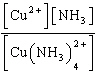b. Kc =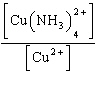c. Kc =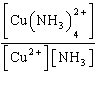d. Kc =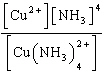e. Kc =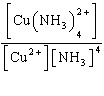4.

Write the expression for K for the reaction below.

Al2S3(s) « 2 Al3+(aq) + 3 S2-(aq)
 a. K = [Al3+]2[S2-]3 b. K = [Al3+][S2-] c. K = [2 Al3+][3 S2-] d. K =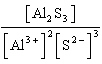e. K =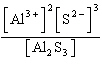5.

Write the expression for Kp for the reaction below.

2 HBr(g) « H2(g) + Br2(l)
 a. Kp =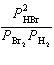b. Kp =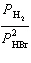c. Kp =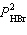d. Kp =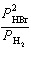e. Kp =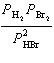6.

Write a balanced chemical equation which corresponds to the following equilibrium constant expression.

Kp =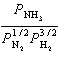a. 1/2 N2(g) + 3/2 H2(g) «NH3(g) b. N2(g) + 3 H2(g) « 2 NH3(g) c. 2 NH3(g) « N2(g) + 3 H2(g) d. NH3(g) « 1/2 N2(g) + 3/2 H2(g) e. 2 N2(g) + 6 H2(g) « 4 NH3(g)

7.

Write a balanced chemical equation which corresponds to the following equilibrium constant expression.

K = [Fe3+][OH-]3
 a. FeOH2+(s) «Fe3+(aq) + OH-(aq) b. 3 Fe3+(aq) + 3 OH-(aq) «3 Fe(OH)3(aq) c. Fe(OH)3(aq) «Fe3+(aq) + 3 OH-(aq) d. Fe(OH)3(s) « Fe3+(aq) + 3 OH-(aq) e. Fe3+(aq) + 3 OH-(aq) « 3 Fe(OH)3(s)

8.

For which one of the following reactions does Kp equal Kc?
 a. 2 SO2(g) + O2(g) « 2 SO3(g) b. 2 O3(g) « 3 O2(g) c. 2 Ag2O(s) «4 Ag(s) + O2(g) d. 2 NH3(g) «3 H2(g) + N2(g) e. CO(g) + NO2(g) « NO(g) + CO2(g)

9.

What is the relationship between Kp and Kc for the reaction below?

CS2(g) + 3 Cl2(g) «S2Cl2(g) + CCl4(g)
 a. Kc =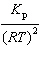b. Kc =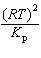c. Kc = (RT)2Kp d. Kc =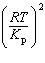e. Kc =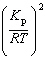10.

Dinitrogen tetraoxide decomposes to nitrogen dioxide.

N2O4(g) « 2 NO2(g)

Calculate the value of Kp, given that Kc = 5.88 ´ 10-3 at 273 K. (R = 0.08206 L·atm/mol·K)
 a. 2.62 ´ 10-4 b. 0.132 c. 7.59 d. 1.70 ´ 102 e. 3.81 ´ 10-3

11.

If the reaction quotient, Q, is greater than Kp, then
 a. the chemical system has reached equilibrium. b. the temperature must be increased for the reaction to proceed in the forward direction. c. the reaction will proceed in the direction that results in fewer gas phase particles. d. the reaction will proceed to the right until equilibrium is established. e. the reaction will proceed to the left until equilibrium is established.

12.

A 4.00 L flask is filled with 0.75 mol SO3, 2.50 mol SO2, and 1.30 mol O2, and allowed to reach equilibrium. Predict the effect on the concentrations of SO3 as equilibrium is achieved by using Q, the reaction quotient. Assume the temperature of the mixture is chosen so that Kc = 12.

2 SO3(g) « 2 SO2(g) + O2(g)
 a. [SO3] will decrease because Q > K. b. [SO3] will decrease because Q < K. c. [SO3] will increase because Q < K. d. [SO3] will increase because Q > K. e. [SO3] will remain the same because Q = K.

13.

Consider the reaction A(g) « 2 B(g) where Kp = 5.0 at 25 °C. If 0.50 mol A and 2.0 mol B are introduced into a 1.0 L flask at 25 °C, what change in concentrations (if any) will occur in time?
 a. [A] will decrease and [B] will decrease. b. [A] will decrease and [B] will increase. c. [A] will increase and [B] will increase. d. [A] will increase and [B] will decrease. e. [A] and [B] remain unchanged.

14.

The reaction below is studied at a high temperature.

PCl5(g) «PCl3(g) + Cl2(g)

At equilibrium, the partial pressures of the gases are as follows: PCl5 = 1.8 ´ 10-2 atm, PCl3 = 5.6 ´ 10-2 atm, and Cl2 = 3.8 ´ 10-4 atm. What is the value of Kp for the reaction?
 a. 3.8 ´ 10-7 b. 1.2 ´ 10-3 c. 3.1 d. 8.5 ´ 102 e. 2.6 ´ 106

15.

Excess Ca(IO3)2(s) is placed in 1.5 L of water. At equilibrium, the solution contains 0.011 M IO3-(aq). What is the equilibrium constant for the reaction below?

Ca(IO3)2(s) « Ca2+(aq) + 2 IO3-(aq)
 a. 3.3 ´ 10-7 b. 6.7 ´ 10-7 c. 1.3 ´ 10-6 d. 5.3 ´ 10-6 e. 6.1 ´ 10-5

16.

At 25 °C, only 1.9 g CaSO4 will dissolve in 2.00 L of water. What is the equilibrium constant for the reaction below?

CaSO4(s) «Ca2+(aq) + SO42-(aq)
 a. 4.9 ´ 10-5 b. 1.9 ´ 10-4 c. 1.4 ´ 10-2 d. 7.0 10-3 e. 0.90

17.

We place 0.0774 mol N2O4(g) in a 1.00 L flask at a given temperature. After reaching equilibrium, the concentration of NO2(g) is 0.0068 M. What is Kc for the reaction below?

N2O4(g)«2 NO2(g)
 a. 8.8 ´ 10-5 b. 5.9 ´ 10-4 c. 6.2 ´ 10-4 d. 7.2 10-4 e. 9.6 ´ 10-2

18.

A sealed tube is prepared with 1.07 atm PCl5 at 500 K. The PCl5 decomposes until equilibrium is established.

PCl5(g) «PCl3(aq) + Cl2(g)

The equilibrium pressure in the tube is 1.54 atm. Calculate Kp.
 a. 0.052 b. 0.20 c. 0.27 d. 0.37 e. 2.2

19.

A mixture of 0.200 mol NO2 and 0.200 mol CO is placed in a 1.00 L flask and given time to equilibrate. Analysis of the equilibrium mixture indicates that 0.134 mol of CO2 is present. Calculate Kc for the reaction.

NO2(g) + CO(g) «NO(g) + CO2(g)
 a. 0.27 b. 0.45 c. 0.67 d. 2.0 e. 4.1

20.

A gaseous mixture of NO2 and N2O4 is in equilibrium. If the concentration of N2O4 is 7.1 ´ 10-4 M, what is the concentration of NO2?

2 NO2(g) « N2O4(g)   Kc = 170
 a. 1.7 ´ 10-11 M b. 4.2 ´ 10-6 M c. 2.0 ´ 10-3 M d. 4.9 ´ 102 M e. 2.4 ´ 105 M

21.

At 25 °C, the decomposition of dinitrogen tetraoxide

N2O4(g) « 2 NO2(g)

has an equilibrium constant (Kp) of 0.144. At equilibrium, the total pressure of the system is 0.48 atm. What is the partial pressure of each gas?
 a. 0.030 atm NO2(g) and 0.42 N2O4(g) b. 0.060 atm NO2(g) and 0.42 N2O4(g) c. 0.16 atm NO2(g) and 0.18 N2O4(g) d. 0.20 atm NO2(g) and 0.28 N2O4(g) e. 0.28 atm NO2(g) and 0.20 N2O4(g)

22.

The equilibrium constant at 25 °C for the dissolution of silver bromide is 5.4 ´ 10-13.

AgBr(s) «Ag+(aq) + Br-(aq)

If an excess quantity of AgBr(s) is added to water and allowed to equilibrate, what is the equilibrium concentration of Ag+?
 a. 2.9 ´ 10-25 M b. 2.7 ´ 10-13 M c. 5.4 ´ 10-13 M d. 1.1 ´ 10-12 M e. 7.3 ´ 10-7 M

23.

Hydrogen iodide can decompose into hydrogen and iodine gases.

2 HI(g) « H2(g) + I2(g) Kp = 0.016

If 0.820 atm HI(g) is sealed in a flask, what is the pressure of each gas when equilibrium is established?
 a. HI = 0.576 atm, H2 = 0.096 atm, I2 = 0.096 atm b. HI = 0.654 atm, H2 = 0.083 atm, I2 = 0.083 atm c. HI = 0.728 atm, H2 = 0.092 atm, I2 = 0.092 atm d. HI = 0.737 atm, H2 = 0.083 atm, I2 = 0.083 atm e. HI = 0.768 atm, H2 = 0.111 atm, I2 = 0.111 atm

24.

We place 1.32 mol PCl5 in a 1.0 L flask and allow it to reach equilibrium at a given temperature. What is the final concentration of Cl2 in the flask?

PCl5(g)« PCl3(aq) + Cl2(g) Kc = 0.47
 a. 0.36 M b. 0.59 M c. 0.62 M d. 0.79 M e. 85 M

25.

The equilibrium constant, Kc, for the following reaction is 1.0 ´ 10-5 at 1500 K.

N2(g) + O2(g) « 2 NO(g)

If 0.750 M N2 and 0.750 M O2 are allowed to equilibrate at 1500 K, what is the concentration of NO?
 a. 6.7 ´ 10-4 M b. 1.2 ´ 10-3 M c. 2.4 ´ 10-3 M d. 2.7 ´ 10-3 M e. 5.5 ´ 10-3 M

26.

Carbonyl bromide decomposes to carbon monoxide and bromine.

COBr2(g) « CO(g) + Br2(g)

Kc is 0.19 at 73 °C. If an initial concentration of 0.63 M COBr2 is allowed to equilibrate, what are the equilibrium concentrations of COBr2, CO, and Br2?
 a. [COBr2] = 0.11 M, [CO] = 0.26 M, [Br2] = 0.26 M b. [COBr2] = 0.28 M, [CO] = 0.35 M, [Br2] = 0.35 M c. [COBr2] = 0.30 M, [CO] = 0.33 M, [Br2] = 0.33 M d. [COBr2] = 0.37 M, [CO] = 0.26 M, [Br2] = 0.26 M e. [COBr2] = 0.63 M, [CO] = 0.35 M, [Br2] = 0.35 M

27.

For the following reaction,

2 SO2(g) + O2(g) «2 SO3(g)

the equilibrium constant, Kp, is 0.758 at 627 °C. What is the equilibrium constant, at 627 °C, for the reaction below?

SO3(g) f SO2(g) + 1/2 O2(g)
 a. 0.660 b. 0.871 c. 1.15 d. 1.32 e. 1.74

28.

The equilibrium constant (Kp) for the following reaction is 3.94 ´ 10-3 at a given temperature.

N2(g) + 2 H2O(g) « 2 NO(g) + 2 H2(g)

What is the equilibrium constant for the reaction below at the same temperature?

3 N2(g) + 6 H2O(g) f 6 NO(g) + 6 H2(g)
 a. 6.12 ´ 10-8 b. 1.31 ´ 10-3 c. 3.94 ´ 10-3 d. 1.18 ´ 10-2 e. 1.58 ´ 10-1

29.

Given the following chemical equilibria,

N2(g) + 3 H2(g) « 2 NH3(g) K1
4 NH3(g) + 5 O2(g) «4 NO(g) + 6 H2O(g) K2
H2(g) + 1/2 O2(g)« H2O(g) K3

Determine the equilibrium constant for the reaction below.

N2(g) + O2(g) f 2 NO(g) K4
 a. K4 = K1 ´ K2 ´ K3 b. K4 = K1 + K2 + K3 c. K4 = K1 +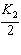+ 3K3 d. K4 =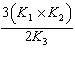e. K4 =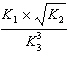30.

Given the following equilibria,

Ni2+(aq) + 2 OH-(aq) « Ni(OH)2(s) K1 = 1.8 ´ 1015
Ni(NH3)62+(aq) « Ni2+(aq) + 6 NH3(aq) K2 = 5.6 ´ 108

determine the equilibrium constant for the following reaction.

Ni(OH)2(s) + 6 NH3(aq) f Ni(NH3)62+(aq) + 2 OH-(aq) K3
 a. 9.9 ´ 10-25 b. 1.8 ´ 10-9 c. 3.1 ´ 10-7 d. 3.2 ´ 106 e. 1.0 ´ 1024

31.

Assume that the following chemical reaction is at equilibrium.

I2(g) + Cl2(g) « 2 ICl(g) DH° = -26.9 kJ

At 25 °C, Kp = 2.0 ´ 105. If the temperature is decreased to 5 °C, which statement applies?
 a. Kp will decrease and the reaction will proceed in the forward direction. b. Kp will decrease and the reaction will proceed in the backward direction. c. Kp will remain unchanged and the reaction will proceed in the forward direction. d. Kp will remain unchanged and the reaction will proceed in the backward direction. e. Kp will increase and the reaction will proceed in the forward direction.

32.

Assume that the following endothermic chemical reaction is at equilibrium.

C(s) + H2O(g) « H2(g) + CO(g)

Which of the following statements are CORRECT?
 1. Increasing the amount of C(s) will increase the equilibrium concentration of CO(g). 2. Increasing the temperature will increase the equilibrium concentration of H2(g). 3. Decreasing the concentration of H2O(g) will increase the equilibrium concentration of CO(g).
 a. 1 only b. 2 only c. 3 only d. 1 and 2 e. 1, 2, and 3

33.

The thermochemical equation for the formation of ammonia from elemental nitrogen and hydrogen is as follows.

N2(g) + 3 H2(g) « 2 NH3(g) DH = -92.2 kJ

Which of the following will drive the equilibrium system to the right?
 a. adding NH3(g) b. removing N2(g) c. increasing the volume of the reaction vessel d. increasing the temperature e. adding H2(g)

34.

In which of the following equilibrium systems will an increase in the pressure have NO effect on the concentrations of products and reactants?
 a. CaO(s) + CO2(g) «CaCO3(s) b. N2(g) + 3 H2(g) « 2 NH3(g) c. H2(g) + Cl2(g) « 2 HCl(g) d. H2(g) + CO2(g) «CO(g) + H2O(l) e. 2 H2O2(g) « 2 H2O(g) + O2(g)

35.

A flask contains the following chemical system at equilibrium.

Ca(OH)2(s) « Ca2+(aq) + 2 OH-(aq)

Addition of which of the following substances will increase the solubility of Ca(OH)2(s)?
 a. HNO3(aq) b. Ca(OH)2(s) c. NaOH(s) d. CaCl2(s) e. Ca(NO3)2(s)